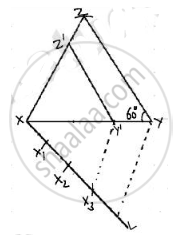Share

# Construct a Triangle Similar to a Given Triangle Xyz with Its Sides Equal to (3/4)Th of the Corresponding Sides of Triangle Xyz. Write the Steps of Construction. - CBSE Class 10 - Mathematics

#### Question

Construct a triangle similar to a given ΔXYZ with its sides equal to (3/4)th of the corresponding sides of ΔXYZ. Write the steps of construction.

#### Solution

let XY = cm, YZ = cm and ∠Y = 60° and then a triangle similar to it whose sides are (3/4)th of the corrosponding sides of ΔXYZ

we follow the following steps to construct triangle.Step of construction

step 1. First of all we draw a line segment XY = 5 cm

step 2. With Y as centre on angle ∠Y = 60°

step 3. with Y as centre and radius YZ = 6 cm, draw on arc

step 4. Join XZ to obtain ΔXYZ

step 5. Below XY1 makes an acute angle ∠YXL = 60°

step 6. Below XL, mark off four points X1, X2, X3 and X4 such that XX1 = X1X2 = X2X3 = X3X4

step 7. Join X4Y

step 8. since we have to construct a triangle each of whose sides is (3/4)th of the corresponding sides of ΔXYZ

So, we take three parts out of four equal parts on XL from point X3 draw X3Y' || X4Y and meeting XY at Y'

step 9. From Y' draw Y'Z' || YZ, and meeting XZ at Z'

Hence, ΔXY'Z' is the required triangle, each of whose sides is (3/4)th of the corresponding sides of ΔXYZ

Is there an error in this question or solution?

#### APPEARS IN

Solution Construct a Triangle Similar to a Given Triangle Xyz with Its Sides Equal to (3/4)Th of the Corresponding Sides of Triangle Xyz. Write the Steps of Construction. Concept: Division of a Line Segment.
S